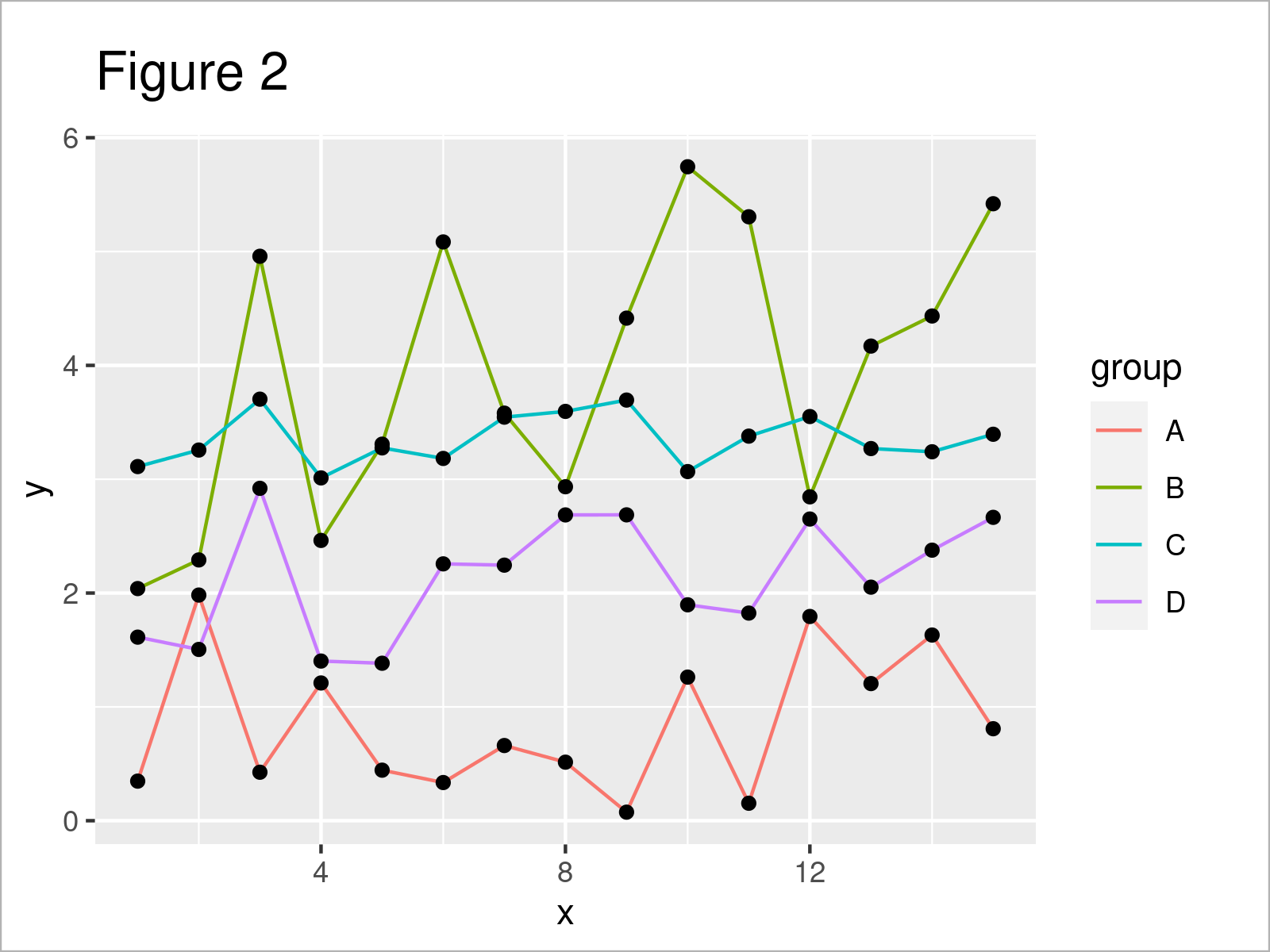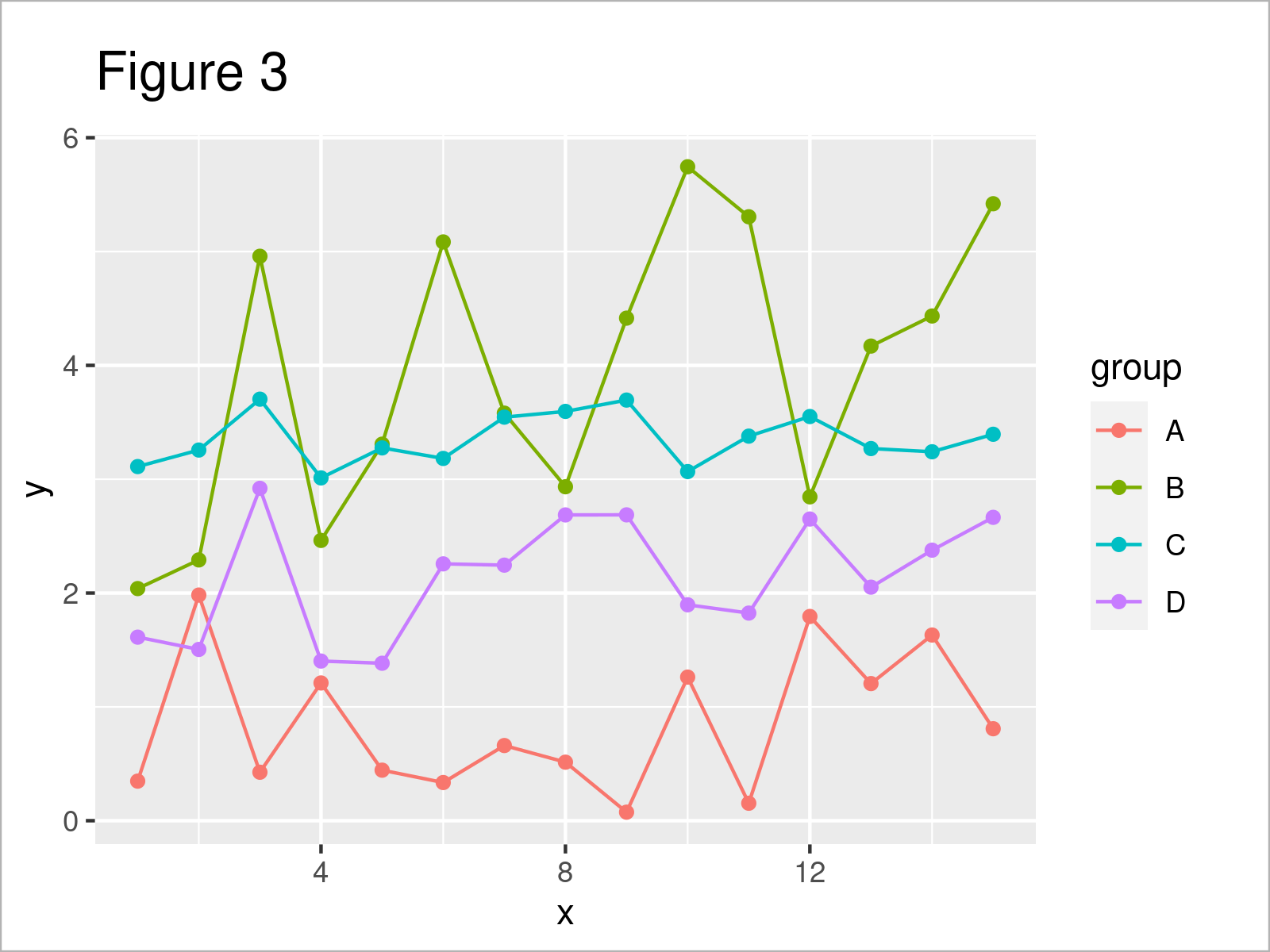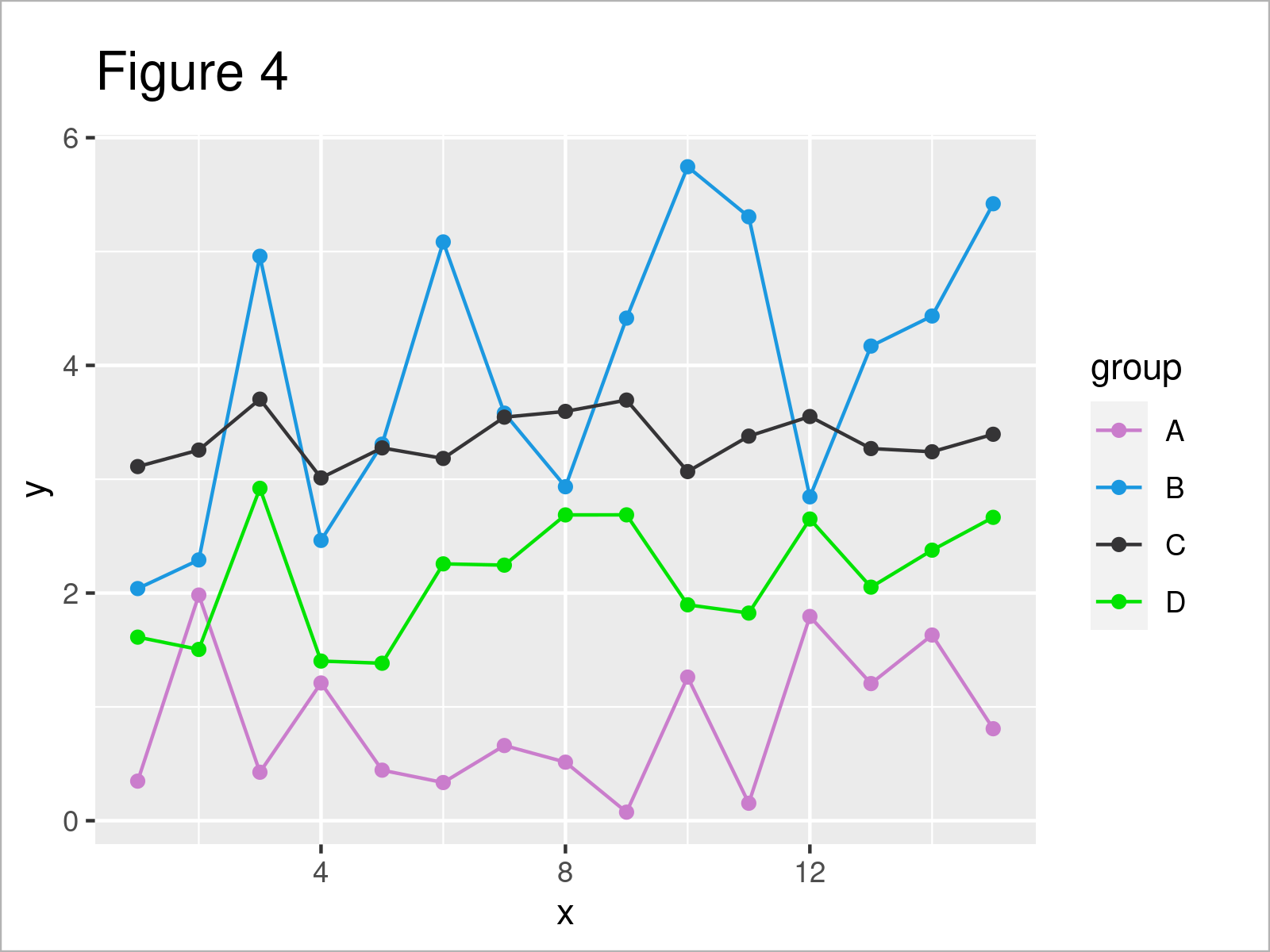# Set Color by Group in ggplot2 Plot in R (4 Examples)

On this page, I’ll show how to specify different group colors in a ggplot2 graph in the R programming language.

The content of the post looks like this:

Let’s dig in:

## Example Data, Packages & Default Plot

As a first step, we’ll need to construct some data that we can use in the examples later on:

```set.seed(68117534)              # Set random seed
data <- data.frame(x = 1:15,    # Create example data frame
y = c(runif(15, 0, 2),
runif(15, 2, 6),
runif(15, 3, 4),
runif(15, 1, 3)),
group = rep(LETTERS[1:4], each = 15))Table 1 shows the first six rows of our example data: it is also shown that the data contains three columns.

For the examples of this tutorial, I’ll also need to install and load the ggplot2 package to RStudio:

```install.packages("ggplot2")     # Install & load ggplot2 package
library("ggplot2")```

As a next step, we can plot our data without colors:

```ggplot(data,                    # Draw ggplot2 plot without colors
aes(x = x,
y = y,
group = group)) +
geom_line() +
geom_point()```By executing the previously shown R programming syntax, we have drawn Figure 1, i.e. a ggplot2 line and point graphic without any colors.

## Example 1: Modify Colors of Single Geom by Group

Example 1 shows how to adjust the colors in a ggplot2 plot by group for a single geom.

In this specific example, we are changing the line colors of our plot:

```ggplot(data,                    # Change colors of lines by group
aes(x = x,
y = y,
group = group)) +
geom_line(aes(col = group)) +
geom_point()```Figure 2 shows the output of the previous syntax: We have adjusted the line colors. However, the color of the points has been kept the same.

## Example 2: Modify Colors of All Geoms by Group

It is also possible to change all colors according to the groups in a data set.

To achieve this, we have to specify the col argument within the aesthetics of the ggplot function:

```ggplot(data,                    # Change colors of lines & points by group
aes(x = x,
y = y,
col = group)) +
geom_line() +
geom_point()```As shown in Figure 3, the previous code has created a new ggplot2 plot where all colors have been changed by group.

## Example 3: Using Manual Color Codes by Group

In this example, I’ll demonstrate how to set manual color codes for each group in a ggplot2 graphic.

For this task, we have to use the scale_color_manual function as shown below. Within this function, we can set a different hex color code for each group:

```ggplot(data,                    # Specify colors manually
aes(x = x,
y = y,
col = group)) +
geom_line() +
geom_point() +
scale_color_manual(values = c("#ca7dcc",
"#1b98e0",
"#353436",
"#02e302"))```## Example 4: Modify Fill Colors of Boxplots by Group

Until now, we have changed the colors in a ggplot2 line and point graph. This example illustrates how to modify the colors of a boxplot graphic.

For this, we can use the fill argument within the aesthetics of the ggplot function:

```ggplot(data,                    # Change colors of boxplots
aes(y = y,
fill = group)) +
geom_boxplot()```By executing the previous code, we have created Figure 5, i.e. several boxplots side-by-side where each box has a different fill color.

## Video, Further Resources & Summary

Have a look at the following video which I have published on my YouTube channel. I explain the examples of this article in the video.

Please accept YouTube cookies to play this video. By accepting you will be accessing content from YouTube, a service provided by an external third party.If you accept this notice, your choice will be saved and the page will refresh.

Furthermore, you might have a look at the other tutorials on my website.

To summarize: You have learned in this article how to set certain group colors in a ggplot2 graphic in the R programming language. In case you have any further questions, don’t hesitate to kindly let me know in the comments section.

Subscribe to the Statistics Globe Newsletter

•Gezahegn Girma
January 10, 2023 11:14 pm

Be blessed brother long live

•January 11, 2023 9:05 am